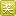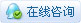232浏览

# [项目分享] 一个DOS模拟器1 创造力 回复本帖可获得 1 创造力奖励! 每人限 1 次(中奖概率 30%)
 [ 本帖最后由 RYAN 于 2022-6-5 12:26 编辑 ]\n\nimport time c = ['POS' , '附件'] fj = ['Calculator' , 'dog zero calculation' , 'Judge Leap Year'] print('Welcome to this operating system') time.sleep(4) print('Appendices:Calculator, dog zero calculation, Judge Leap Year') while 1:     ram = input('>>>')     if ram == fj:         a = input('Enter A to power, enter B to close the calculator, enter C to multiply:')         if a != 'A' and a != 'C' and a != 'B':             print('TAT\n Invalid input \nTAT')         elif a == 'A' :             a = float(input('Please enter the first number:'))             b = float(input('Please enter the second number:'))             c = a**b             c = str(c)             print('Equal to'+c)         elif a == 'C' :             a = float(input('Please enter the first number:'))             b = float(input('Please enter the second number:'))             print('Equal to' , a*b)         else:             print('out')             break     elif ram == fj:         j = int(input('How old is the dog:'))         if j <= 0 :             print('That’s not possible')         elif j == 1 :             print('The equivalent of 14 years old')         elif j == 2 :             print('The equivalent of 22 years old')         else:             h = (j+22)*2             print('The equivalent of' , h , 'years old')     elif ram == fj:         n = int(input('Please enter a year:'))         if n % 4 == 0 and n % 100 != 0 or n % 400 == 0:             print('{0}year, is a leap year.'.format(n))         else:             print('{0}year, not leap year.'.format(n))     else:         print('Invalid input')### RYAN见习技师 楼主|

 快来看，快来看，快来看！

 yyds,666 快来看，快来看，快来看

 您需要登录后才可以回帖 登录 | 立即注册 本版积分规则 回帖并转播 回帖后跳转到最后一页

[[wsData.name]]

#### 硬件清单

• [[d.name]]#### 楼主的其它帖子